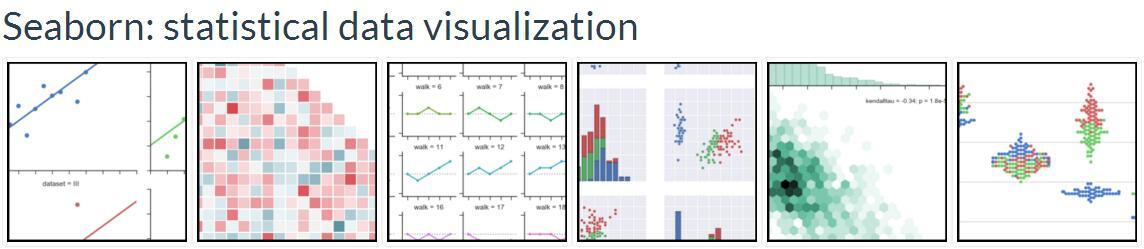## 第一章 艺术化的图表控制

Matplotlib无疑是高度可定制的，但快速实施出吸引人的细节就变得有些复杂。Seaborn作为一个带着定制主题和高级界面控制的Matplotlib扩展包，能让绘图变得更轻松，本部分主要介绍seaborn是如何对matplotlib输出的外观进行控制的。

``````%matplotlib inline
#jupyter notebook 中的魔法函数，如果不是使用该软件请使用plt.show()用于显示图像
import numpy as np
import matplotlib as mpl
import matplotlib.pyplot as plt
np.random.seed(sum(map(ord,"aesthetics")))  # 定义种子``````

``````def sinplot(flip=1):
x = np.linspace(0,14,100)
for i in range(1,7):
plt.plot(x,np.sin(x+i*.5)*(7-i)*flip)``````

``sinplot()``

``````import seaborn as sns
sinplot()``````

seaborn默认的灰色网格底色灵感来源于matplotlib却更加柔和。大多数情况下，图应优于表。seaborn的默认灰色网格底色避免了刺目的干扰，对于多个方面的图形尤其有用，是一些更复杂的工具的核心。

Seaborn将matplotlib参数分成两个独立的组。第一组设定了美学风格，第二组则是不同的度量元素，这样就可以很容易地添加到代码当中了。

## 样式控制：axes_style() and set_style()

• darkgrid 黑色网格（默认）
• whitegrid 白色网格
• dark 黑色背景
• white 白色背景
• ticks 应该是四周都有刻度线的白背景？

``````sns.set_style("whitegrid")
data = np.random.normal(size=(20, 6)) + np.arange(6) / 2
sns.boxplot(data=data);``````

``````sns.set_style("dark")
sinplot()``````

``````sns.set_style("white")
sinplot()``````

``````sns.set_style("ticks")
sinplot()
# 官方的例子在上方/右方也拥有刻度线，而验证时却没有（是jupyter notebook的原因？）``````

## 用despine()进行边框控制

white和ticks参数的样式，都可以删除上方和右方坐标轴上不需要的边框，这在matplotlib中是无法通过参数实现的，却可以在seaborn中通过despine()函数轻松移除他们。

``````sns.set_style("white")
sinplot() # 默认无参数状态，就是删除上方和右方的边框
sns.despine()``````

``````f, ax = plt.subplots()
sns.violinplot(data=data)
sns.despine(offset=10, trim=True); # offset 两坐标轴离开距离；``````

``````sns.set_style("whitegrid")
sns.boxplot(data=data, palette="deep")
sns.despine(left=True) # 删除左边边框
st = sns.axes_style("darkgrid")``````

despine(fig=None, ax=None, top=True, right=True, left=False, bottom=False, offset=None, trim=False)

## 临时设定图形样式

``````with sns.axes_style("darkgrid"):
plt.subplot(211)
sinplot()
plt.subplot(212)
sinplot(-1)``````

## seaborn样式中最重要的元素

``````sns.axes_style()

{'axes.axisbelow': True,
'axes.edgecolor': 'white',
'axes.facecolor': '#EAEAF2',
'axes.grid': True,
'axes.labelcolor': '.15',
'axes.linewidth': 0.0,
'figure.facecolor': 'white',
'font.family': ['sans-serif'],
'font.sans-serif': ['Arial',
'Liberation Sans',
'Bitstream Vera Sans',
'sans-serif'],
'grid.color': 'white',
'grid.linestyle': '-',
'image.cmap': 'Greys',
'legend.frameon': False,
'legend.numpoints': 1,
'legend.scatterpoints': 1,
'lines.solid_capstyle': 'round',
'text.color': '.15',
'xtick.color': '.15',
'xtick.direction': 'out',
'xtick.major.size': 0.0,
'xtick.minor.size': 0.0,
'ytick.color': '.15',
'ytick.direction': 'out',
'ytick.major.size': 0.0,
'ytick.minor.size': 0.0}``````

``````sns.set_style("darkgrid", {"axes.facecolor": ".9"})
sinplot()``````

## 通过 plotting_context() 和 set_context() 调整绘图元素

``sns.set()``

``````sns.set_context("paper")
plt.figure(figsize=(8,6))
sinplot()``````

``````# default 默认设置
sns.set_context("notebook")
plt.figure(figsize=(8,6))
sinplot()``````

``````sns.set_context("talk")
plt.figure(figsize=(8,6))
sinplot()``````

``````sns.set_context("poster")
plt.figure(figsize=(8,6))
sinplot()``````

``````sns.set_context("notebook", font_scale=1.5, rc={"lines.linewidth": 2.5})
sinplot()``````

【第一章 完】

【未禾原创，所有章节已经完结，其他章节请点击跳转】

Seaborn(sns)官方文档学习笔记（第一章 艺术化的图表控制） - 知乎专栏

Seaborn(sns)官方文档学习笔记（第二章 斑驳陆离的调色板） - 知乎专栏

Seaborn(sns)官方文档学习笔记（第三章 分布数据集的可视化） - 知乎专栏

Seaborn(sns)官方文档学习笔记（第四章 线性关系的可视化） - 知乎专栏

Seaborn(sns)官方文档学习笔记（第五章 分类数据的绘制） - 知乎专栏

Seaborn(sns)官方文档学习笔记（第六章 绘制数据网格） - 知乎专栏